Get instant live expert help with Excel or Google Sheets“My Excelchat expert helped me in less than 20 minutes, saving me what would have been 5 hours of work!”

#### Post your problem and you’ll get Expert help in seconds.

Your message must be at least 40 characters
Our professional Expert are available now. Your privacy is guaranteed.

# Learn How to Use the Excel GCD Function

GCD function stands for Greatest Common Divisor. this function allows us to get the greatest divisor which is common for two or more numbers. It is the largest integer that can divide all the given numbers of a database without reminders. In this tutorial, we will learn how to use the GCD function in Excel.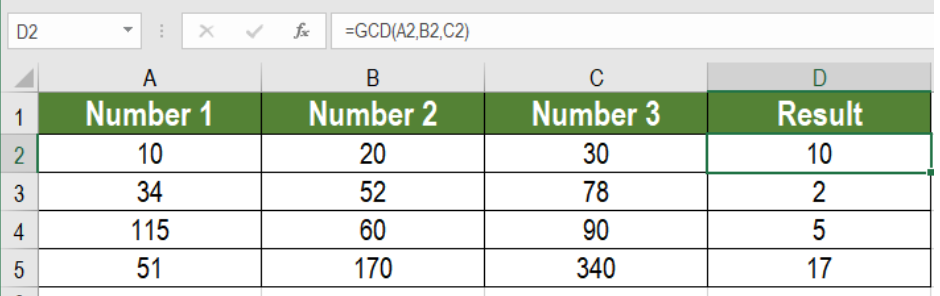Figure 1: Example of How to Use the GCD Function in Excel

## The purpose of GCD function

The main purpose of this function is to extract the biggest common number. This is the number which can divide all the numbers provided as arguments.

## Syntax

`=GCD ( number, [number 2],…)`

• [Number 1]
Required. This is the first number that we want find the divisor for.
• [Number 2…]
Optional. This is the argument for the subsequent numbers. Excel truncates the numbers if they are not integers.

## How the GCD Function Works

The table has two numbers 66 and 99 in column A and B. To extract the biggest divisor number by using GCD, we need to apply the formula,

`=GCD(A2:C2)`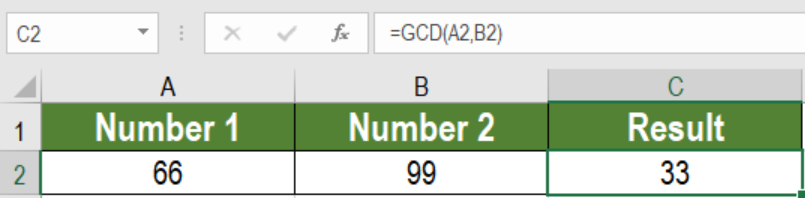Figure 2: The GCD Function in action

The result shows 33. Other numbers like 3 and 11 can divide these two numbers. But, 33 is the largest number which can divide the numbers.

## Example

The following datasets contains numbers in column A, B and C. To find the greatest common divisor for each row:

• We need to go to cell D2 and click on it with the mouse.
• Next, we need to click on Formulas>Insert Function. In the search box type GCD and click Go.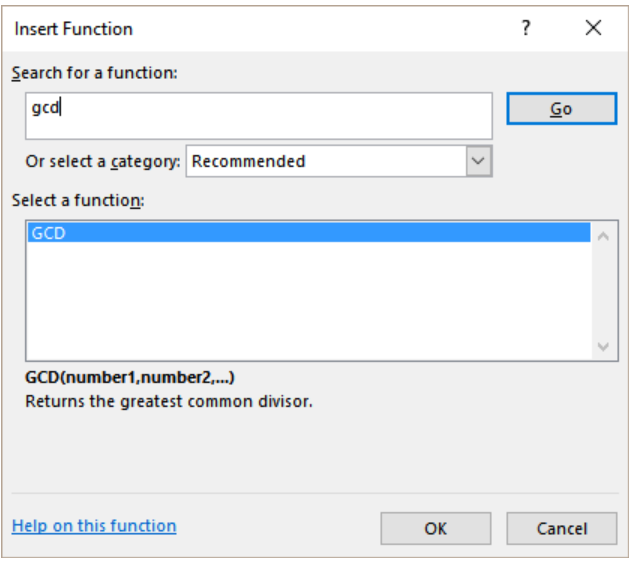Figure 3: Example of the Insert Function Window

• From the select a function box, we need to select GCD and press Ok.
• Then, we need to choose the cells containing the numbers for the arguments Number1, Number2 and Number3. These will be the numbers 10, 20 and 30.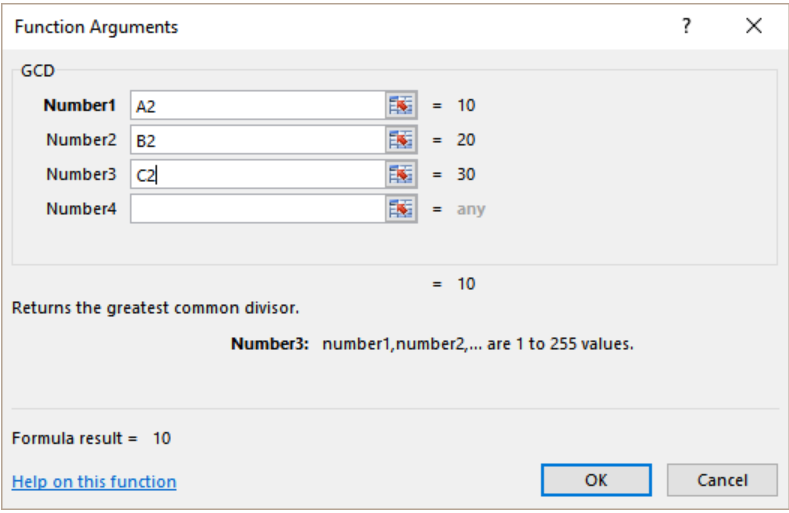Figure 4: Example of Setting the Parameters of the GCD Function

• Lastly, Clicking Ok will give the results. Next we need to drag the formula using the fill handle from cells D2 to D5..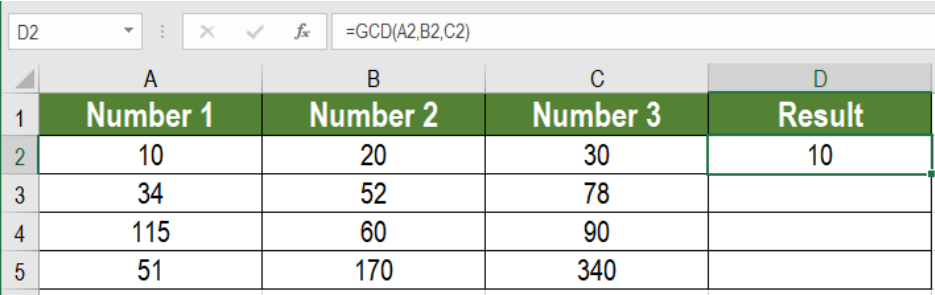Figure 5: Example of Applying the GCD function to the Dataset

The outcome will be 10. Here 10 is the biggest common number which can divide all three numbers.

The GCD function in Excel is not very common and few people use it while working on excel. But the formula is very effective.

## Notes

• The GCD function can take 255 numbers as arguments.
• The GCD function returns a #VALUE error if the arguments provided are not valid numbers.

Most of the time, the problem you will need to solve will be more complex than a simple application of a formula or function. If you want to save hours of research and frustration, try our live Excelchat service! Our Excel Experts are available 24/7 to answer any Excel question you may have. We guarantee a connection within 30 seconds and a customized solution within 20 minutes.